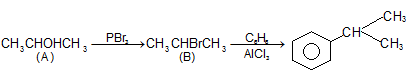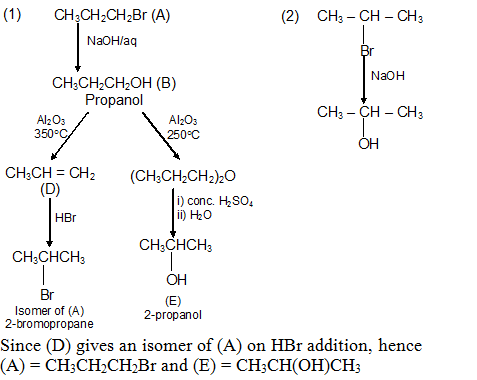Click to Chat

1800-1023-196

+91-120-4616500

CART 0

• 0

MY CART (5)

Use Coupon: CART20 and get 20% off on all online Study Material

ITEM
DETAILS
MRP
DISCOUNT
FINAL PRICE
Total Price: Rs.

There are no items in this cart.
Continue Shopping• Complete JEE Main/Advanced Course and Test Series
• OFFERED PRICE: Rs. 15,900
• View Details

```Solved Examples on Alcohols, Phenols and Ether:

Question:1, Compound (A) gives positive Lucas test in 5 minutes when 6.0 gm of (A) is treated with Na metal, 1120 ml of H2 is evolved at STP. Assuming (A) to contain one of oxygen per molecule, write structural formula of (A). Compound (A) when treated with PBr3 gives (B) which when treated with benzene in prsence of anhydrous AlCl3 gives (C). What are (B) and (C)?

Solution:

i) A gives Lucas test within 5 min. and thus (A) is secondary alcohol

ii) (A) contains one O-atom and thus, only one O-H group in (A).

Thus, (A) is CnH2n+1 OH.

iii) Since, 1120 ml of H2 is given by the action of Na over 6 gm (A)

11200 ml H2 is given by the action of Na = (6×11200)/1120  = 60 gm (A)

M.wt. of (A) is 60

Since one mole of an alcohol having one OH group gives 11200 ml H2 at STP with Na.

iv) CnH2n+1Oh = 60
n = 3
or (A) in C3H7OH; being secondary alcohol its formula is CH3.CHOH.CH3.

Reactions Involved:Question:2, 0.396 gm of a bromoderivative of a hydrocarbon (A) when vapourised occupied 67.2 ml at NTP. On reaction with aqueous NaOH (A) gives (B). (B) when passed over alumina at 250°C gives a neutral compound (C), while at 350°C it gives a hydrocarbon (D), (D) when heated with HBr gives an isomer of (A). When (D) is treated with conc. H2SO4 and the product is diluted with water and distilled, (E) is obtained. Identify (A) to (E) and explain the reactions involved.

Solution: Molecular weight of A = (0.369 ×22.1×1000)/67.2

(A) is a bromiderivative so it may be written and R – Br

MRBr = 123, So, R = 43 Q Br = 80 (Atomic weight)

i.e. Cn H2n + 1 = 43 or, 12n + 2n + 1 = 43

or n = 3

A = C3H7Br

So, the possible structure of (A) are CH3CH2CH2Br or CH3CH(Br)CH3

The reactions are as follows.Question:3. Explain the lower boiling point and decreased water solubility of o-nitrophenol and o-hydroxybenzaldehyde as compared with their m-and p-isomers.

Solution: Intramolecular H–bonding (chelation) in the o-isomers inhibits intermolecular attraction, lowering the boiling point, and reduces H-bonding with H2O, decreasing water solubility. Intramolecular chelation cannot occur in m- and p-isomers.```### Course Features

• 728 Video Lectures
• Revision Notes
• Previous Year Papers
• Mind Map
• Study Planner
• NCERT Solutions
• Discussion Forum
• Test paper with Video Solution